# Types of Fractions

In Maths, there are three major types of fractions. They are proper fractions, improper fractions and mixed fractions. Fractions are those terms which have numerator and denominator. Based on these two terms we define its types.

Fractions are the terms used to determine the parts of a whole object. For example, a pizza is divided into four pieces, so each piece of it is represented as 1/4th of the pizza. Here 1 is the numerator and 4 is the denominator.

Based on numerator and denominator, apart from these three major types of fractions, there are three more types of fractions namely like & unlike fractions and equivalent fractions. Hence, there are in total six types of fractions such as:

Note: The first three fractions are defined for a single fraction but the other three fractions, determine the comparison between two or more fractions.

In real-life scenarios all the measured quantities cannot be an absolute whole number, we may have to deal with parts and portions of whole things, and this is where the concept of fraction comes into the picture.

## Different Types of Fraction

A fraction is considered to be the ratio of two numbers. The upper number is called Numerator, and the lower part is known as the Denominator. When a whole of something is divided into the number of parts, then each part is referred to as a fraction. A typical example of how the concept of fraction works is illustrated in the below figure.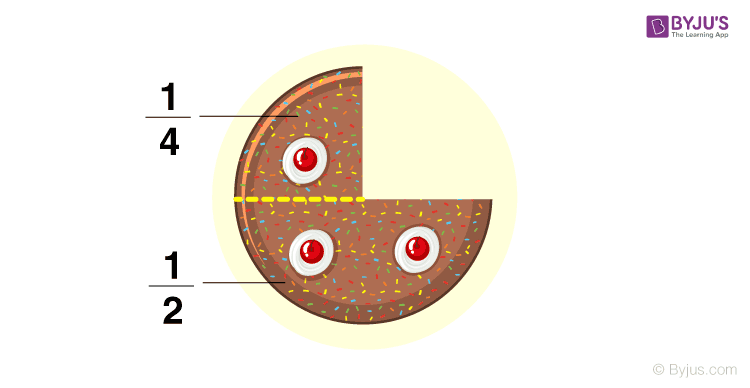Consider another example, a WHOLE pizza is divided into EIGHT PIECES that form the fraction, where one piece of the whole pizza is represented as 1/8, where 1 is the numerator and 8 is the denominator.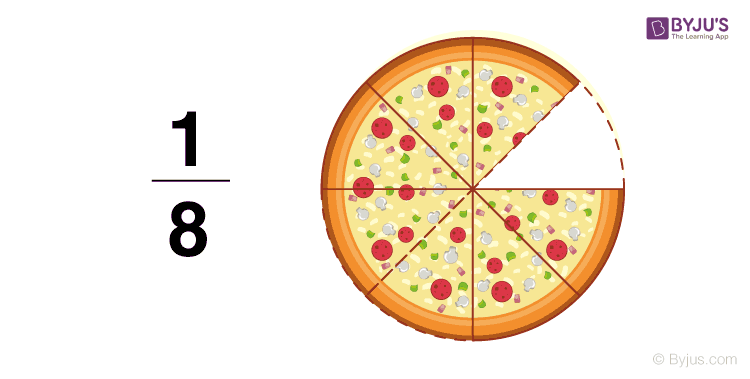### Proper Fraction

A fraction where the numerator is less than the denominator, then it is known as a proper fraction.

i.e., Numerator < Denominator

For example,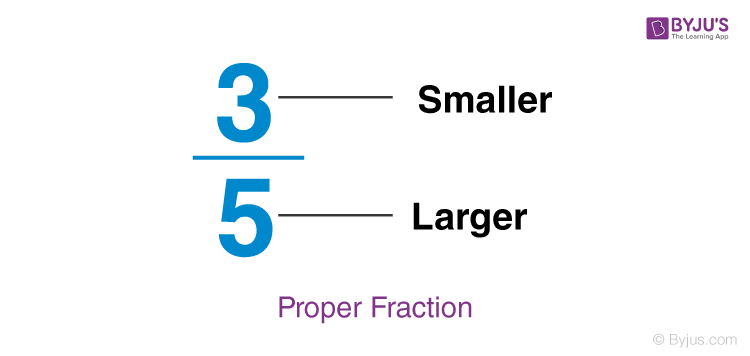Note:

• The value of proper fraction after further simplification is always less than 1.

### Improper Fraction

A fraction where the numerator is greater than the denominator, then it is known as an improper fraction.

i.e., Numerator > Denominator

For example,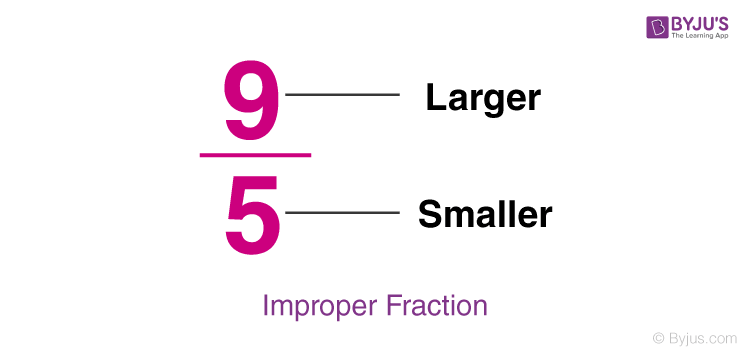Note:

• All the natural numbers can be represented in the form of fractions, where the denominator is always equal to 1.
• The simplification of improper fraction results in the value which is equal or greater than 1, but not less than 1.

### Mixed Fraction

A mixed fraction is the combination of a natural number and fraction. It is basically an improper fraction.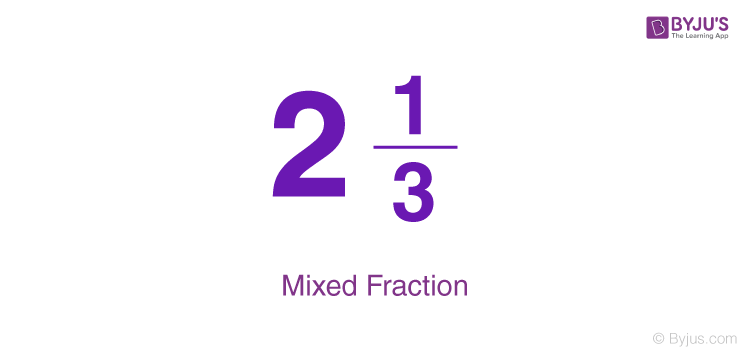Note:

• Mixed fractions can always be converted into a fraction.
• An improper fraction can be converted into a mixed fraction.
• A mixed fraction is always greater than 1.

### Like Fractions

The fractions which have the same denominators are called like fractions.

For example 1/2, 3/2, 5/2, 7/2 are like fractions.

The simplification of such fractions is easy, as all the denominators here are the same. Suppose we need to add all the above like fractions, then;

1/2 + 3/2 + 5/2 + 7/2 = (1+3+5+7)/2 = 16/2 = 8

### Unlike Fractions

The fractions which have unequal denominators or different denominators are called, unlike fractions.

For example 1/2, 1/3, 1/4, 1/5, are unlike fractions.

Simplication for such fractions is a little lengthy method since we need to factorise the denominator first and then simplify them (in case of addition and subtraction).

• Suppose, we have to add 1/2 and 1/3. Then first we will find the LCM of 2 and 3 which is equal to 6.
• Now we need to multiply 1/2 by 3 and 1/3 by 2, both in numerator and denominator.
• The fractions become 3/6 and 2/6.
• Now if we add 3/6 and 2/6, we get;
• 3/6+2/6 = 5/6

### Equivalent Fractions

When two or more fractions have the same result after simplification for which they represent the same portion of the whole, then such fractions are equal to each other and are called equivalent fractions.

For example, 1/2 and 2/4 are equivalent.

1/3 and 3/9 are equivalent.

## Examples

Let us see some examples here based on the fraction’s types.

• Examples of Proper Fractions:  2/3, 2/4, 2/5, 1/2. 4/7, 7/9, etc. (Numerator < Denominator)
• Examples of Improper Fractions: 3/2, 4/2, 5/2, 7/4, 9/7, 8/5, etc. (Numerator > Denominator)
• Examples of Mixed Fractions: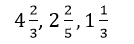etc. (Combination of whole numbers, proper and improper fractions)
 Related Links Fractions Worksheet How To Simplify Fractions Improper Fractions Like Fractions Unlike Fractions Mixed Fractions Partial Fractions

### Video Lesson## Frequently Asked Questions – FAQs

Q1

### What are the types of fractions?

The three major types of fractions are:
Proper fraction
Improper fraction
Mixed fraction
Q2

### What is a proper fraction? Give an example.

A proper fraction is a fraction which has its numerator value less than the denominator. For example, ⅔, 6/7, 8/9, etc. are proper fractions.
Q3

### What are the six kinds of fractions?

The six kinds of fractions are, proper fractions, improper fractions, mixed fractions, like fractions, unlike fractions and equivalent fractions.
Q4

### Explain improper fraction with example.

An improper fraction has its numerator greater than the denominator. The examples are 12/5, 11/3, 9/2,10/7, etc.
Q5

### What are like fractions and unlike fractions?

When two fractions have the same denominator, then they are said to be like fractions. For example, 3/2 and 5/2 are like fractions, and we can perform addition and subtraction operations on them. Such as:
3/2 + 5/2 = (3+5)/2 = 8/2 = 4
When two fractions have different denominators, then they are said to be unlike fractions. For example, 3/2 and 4/3 are improper fractions and we need to rationalise the denominators to perform addition and subtraction.
3/2 + 4/3 = (3×3)/(2×3) + (4×2)/(3×2)
= (9/6)+(8/6)
= (9+8)/6 = 17/6
Test your knowledge on Types Of Fraction

1. Sir/Ma’am, I do like the fact that you share information online, and most of what you share are are right, but please if we can make it all correct, we must do. There are just some parts that have to be verified and edited. But all in all, it was a great job and please don’t sop educating people. Good luck Sir/Ma’am!!!

• Thank you mam

2. This all is informative but if u had added some question methods it can be so easy. Anyway it was a good idea, thank u

3. Thanks you.

4. thank you for the fractions

5. Hi, mam the video I learnd and I passed math exam thank you mam

6. Very nice

7. Thank you Byjus team for this nice information one’s again thank you